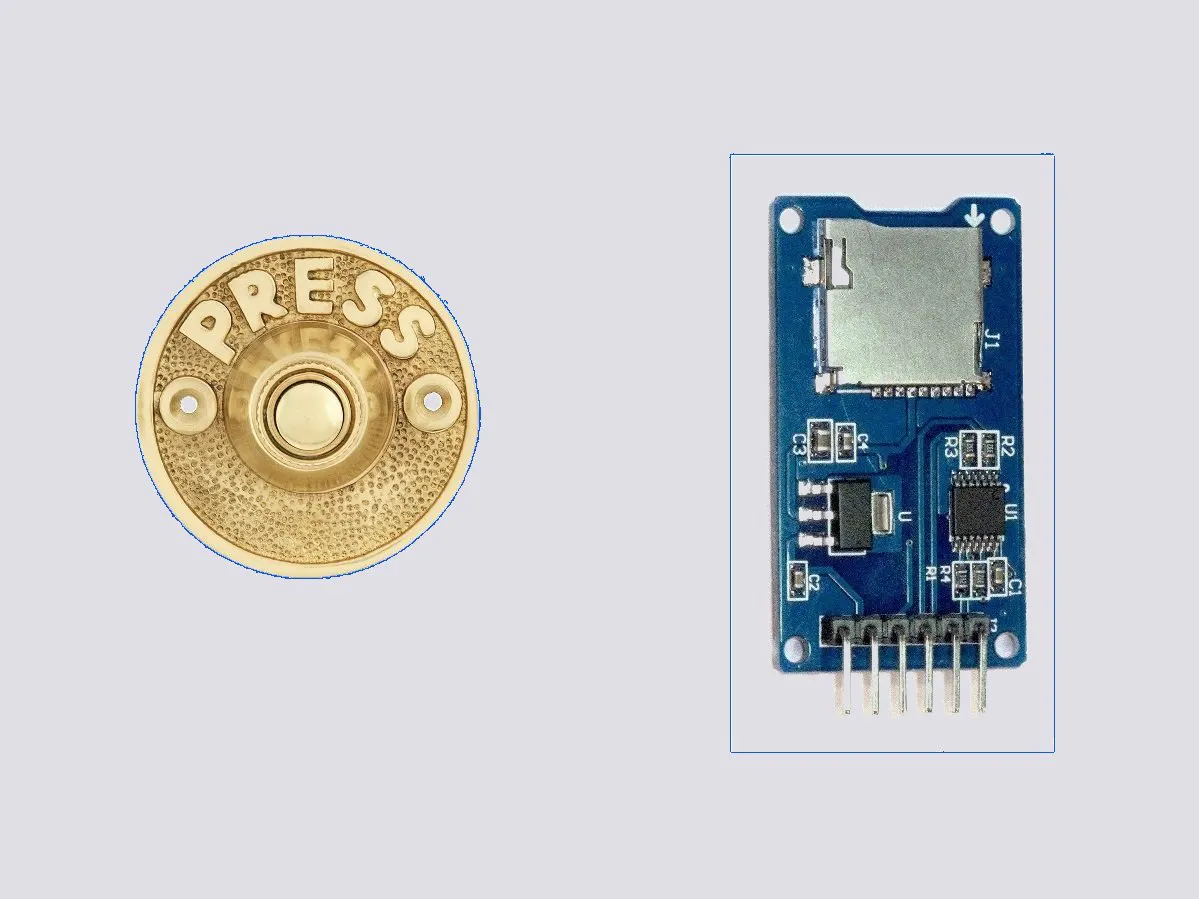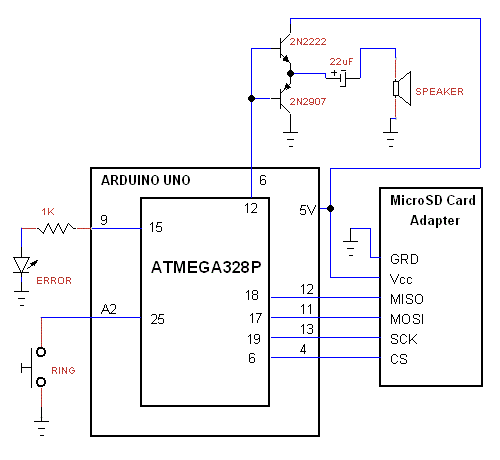Project tutorial# SD Card Doorbell

Doorbell plays sound from microSD card and Arduino.

• 4,485 views
• 10 respects

## Components and supplies

The firmware works for SD or SDHC cards only. Do not use SDXC cards with this project because some of them work on 1.8V drive. The code detects whether the card is SD or SDHC and selects the proper addressing system for the card. The quality of the audio is reasonable.

The software has the functions needed to read SD/SDHC card formatted in FAT32. The software can play only PCM 22.050KHz, 8 bits, mono. The bytes from the file are streamed to PWM generator and with an external low pass filter you get the audio. The PWM gives 64KHz wave with duty cycle modulated to the audio amplitude. A low pass filter removes the 64KHz component.

SD card interfaces the microcontroller in SPI mode. Reading data is in multi-blocks. The Error LED indicates error of communication with the SD card.

The software searches for files entries in the root directory only.

For more details visit my website http://moty22.co.uk/bell.php

TO SET UP THE CARD:

• Create sound file type .wav
• Name the file with short name, 8 low case characters max.
• Save the file as 22.050KHz, 8 bits, mono.
• Format the card with FAT32.
• Add the files to the root directory of the card (don't use a subdirectory).

## Code

##### SD doorbellC/C++
```/*
* sd_bell.ino
*
* Created: 5/06/2017 23:34:47
*  Author: mfprojects.co.uk
*/

const int CS = 4;          // SD CS connected to digital pin PD4
const int mosi = 11;
const int clk = 13;
const int slave = 10;          // Slave Select
const int ampON = 8;          // led connected to digital pin PB0
const int ErrorLED = 9;          // error led connected to digital pin PB1
const int audio = 6;     //output D6
const int ring = A2;     //ring pushbutton
int ringPB=1;         // ring pushbutton status

unsigned long loc,BootSector, RootDir, SectorsPerFat, RootDirCluster, DataSector, FileCluster, FileSize;  //
unsigned int BytesPerSector, ReservedSectors, card;
unsigned char fn=1, sdhc=0, SectorsPerCluster, Fats;

void setup() {
// put your setup code here, to run once:
pinMode(CS, OUTPUT);
pinMode(mosi, OUTPUT);
pinMode(clk, OUTPUT);
pinMode(ampON, OUTPUT);
pinMode(ErrorLED, OUTPUT);
pinMode(slave, OUTPUT);          // set spi to master
//pinMode(audio, OUTPUT);
pinMode(ring, INPUT_PULLUP);

//spi init
SPCR = _BV(SPE) | _BV(MSTR) | _BV(SPR0) | _BV(SPR1);  // Enable spi, Master, set clock rate fck/64
SPSR = _BV(SPI2X);    //set clock rate fck/64 = 250KHz

//PWM Timer0
OCR0A = 64;
TCCR0A = _BV(COM0A1) | _BV(WGM01) | _BV(WGM00);  //output in phase, fast PWM mode
TCCR0B = _BV(CS00); // 16MHz/256 = 64KHz
pinMode(audio, OUTPUT);

//50us, 20KHz timer2
OCR2A = 45;
TCCR2A = _BV(WGM21); //CTC mode
TCCR2B = _BV(CS21); // set prescaler to 8 and start the timer

digitalWrite(CS, HIGH);
digitalWrite(ampON, LOW);
digitalWrite(ErrorLED, LOW);

InitSD();
if(sdhc){card = 1; fn=0;}else{card=512;}  //SD or SDHC
fat();
if(BytesPerSector!=512){digitalWrite(ErrorLED, HIGH);}

}

void loop()
{
if(ringPB==LOW)
{
file(fn*32+20,0);    //32 bytes per file descriptor at offset of 20

loc=(1 + (DataSector) + (unsigned long)(FileCluster-2) * SectorsPerCluster) * card ;
digitalWrite(ampON, HIGH);
digitalWrite(ampON, LOW);
}

}

{
unsigned int i,r;
digitalWrite(CS, LOW);
if(r != 0)digitalWrite(ErrorLED, HIGH);     //if command failed

while(FileSize--)
{
while(spi(0xFF) != 0xFE); // wait for first byte
for(i=0;i<512;i++){
OCR2A = 45;   //20KHz
while (!(TIFR2 & _BV(OCF2A))){}         // wait for the overflow event

OCR0A=spi(0xFF);    //write byte to PWM
TIFR2 |= _BV(OCF2A);  //TMR2 interrupt reset

}
spi(0xFF);
}

Command(12,0x00,0xFF);  //stop transmit
spi(0xFF);
spi(0xFF);
digitalWrite(CS, HIGH);
spi(0xFF);
}

void file(unsigned int offset, unsigned char sect)  //find files
{
unsigned int r,i;
unsigned char fc, fs; //

digitalWrite(CS, LOW);
r = Command(17,(RootDir+sect)*card,0xFF);   //read boot-sector for info from file entry
if(r != 0)digitalWrite(ErrorLED, HIGH);     //if command failed

while(spi(0xFF) != 0xFe); // wait for first byte
for(i=0;i<512;i++){
if(i==offset){fc=spi(0xFF);}
else if(i==offset+1){fc=spi(0xFF);}
else if(i==offset+6){fc=spi(0xFF);}
else if(i==offset+7){fc=spi(0xFF);}

else if(i==offset+8){fs=spi(0xFF);}
else if(i==offset+9){fs=spi(0xFF);}
else if(i==offset+10){fs=spi(0xFF);}
else if(i==offset+11){fs=spi(0xFF);}
else{spi(0xFF);}

}
spi(0xFF);
digitalWrite(CS, HIGH);
spi(0xFF);
FileCluster = fc | ( (unsigned long)fc << 8 ) | ( (unsigned long)fc << 16 ) | ( (unsigned long)fc << 24 );
FileSize = fs | ( (unsigned long)fs << 8 ) | ( (unsigned long)fs << 16 ) | ( (unsigned long)fs << 24 );
FileSize = FileSize/512-1;    //file size in sectors
}

void fat (void)
{
unsigned int r,i;
unsigned char pfs,bps1,bps2,rs1,rs2,spf,rdc; //pfs=partition first sector ,de1,de2,spf1,d

digitalWrite(CS, LOW);
if(r != 0)digitalWrite(ErrorLED, HIGH);     //if command failed

while(spi(0xFF) != 0xFe); // wait for first byte
for(i=0;i<512;i++){
if(i==454){pfs=spi(0xFF);} //pfs=partition first sector
else if(i==455){pfs=spi(0xFF);}
else if(i==456){pfs=spi(0xFF);}
else if(i==457){pfs=spi(0xFF);}
else{spi(0xFF);}

}
spi(0xFF);
digitalWrite(CS, HIGH);
spi(0xFF);
//convert 4 bytes to long int
BootSector = pfs | ( (unsigned long)pfs << 8 ) | ( (unsigned long)pfs << 16 ) | ( (unsigned long)pfs << 24 );

digitalWrite(CS, LOW);
if(r != 0)digitalWrite(ErrorLED, HIGH);     //if command failed

while(spi(0xFF) != 0xFe); // wait for first byte
for(i=0;i<512;i++){

if(i==11){bps1=spi(0xFF);} //bytes per sector
else if(i==12){bps2=spi(0xFF);}
else if(i==13){SectorsPerCluster=spi(0xFF);}
else if(i==14){rs1=spi(0xFF);}
else if(i==15){rs2=spi(0xFF);}
else if(i==16){Fats=spi(0xFF);} //number of FATs
else if(i==36){spf=spi(0xFF);}
else if(i==37){spf=spi(0xFF);}
else if(i==38){spf=spi(0xFF);}
else if(i==39){spf=spi(0xFF);}
else if(i==44){rdc=spi(0xFF);}
else if(i==45){rdc=spi(0xFF);}
else if(i==46){rdc=spi(0xFF);}
else if(i==47){rdc=spi(0xFF);}
else{spi(0xFF);}

}
spi(0xFF);
digitalWrite(CS, HIGH);
spi(0xFF);

BytesPerSector = bps1 | ( (unsigned int)bps2 << 8 );
ReservedSectors = rs1 | ( (unsigned int)rs2 << 8 ); //from partition start to first FAT
RootDirCluster = rdc | ( (unsigned long)rdc << 8 ) | ( (unsigned long)rdc << 16 ) | ( (unsigned long)rdc << 24 );
SectorsPerFat = spf | ( (unsigned long)spf << 8 ) | ( (unsigned long)spf << 16 ) | ( (unsigned long)spf << 24 );
DataSector = BootSector + (unsigned long)Fats * (unsigned long)SectorsPerFat + (unsigned long)ReservedSectors;  // + 1
RootDir = (RootDirCluster -2) * (unsigned long)SectorsPerCluster + DataSector;
}

unsigned char spi(unsigned char data)   // send character over spi
{
SPDR = data;  // Start transmission
while(!(SPSR & _BV(SPIF)));   // Wait for transmission to complete

}
//assemble a 32 bits command
char Command(unsigned char frame1, unsigned long adrs, unsigned char frame2 )
{
unsigned char i, res;
spi(0xFF);
spi((frame1 | 0x40) & 0x7F);  //first 2 bits are 01
spi(frame2 | 1);        //CRC and last bit 1

for(i=0;i<10;i++) // wait for received character
{
res = spi(0xFF);
if(res != 0xFF)break;
}
return res;
}

void InitSD(void)
{
unsigned char i,r;

digitalWrite(CS, HIGH);
for(i=0; i < 10; i++)spi(0xFF);   // min 74 clocks
digitalWrite(CS, LOW);      // Enabled for spi mode

i=100;  //try idle state for up to 100 times
while(Command(0x00,0,0x95) !=1 && i!=0)
{
digitalWrite(CS, HIGH);
spi(0xFF);
digitalWrite(CS, LOW);
i--;
}
if(i==0)  digitalWrite(ErrorLED, HIGH); //idle failed

if (Command(8,0x01AA,0x87)==1){         //check card is 3.3V
r=spi(0xFF); r=spi(0xFF); r=spi(0xFF); r=spi(0xFF);   //rest of R7
if ( r == 0x01 && r == 0xAA ){    //Vdd OK (3.3V)

//Command(59,0,0xFF);   //CRC off
Command(55,0,0xFF);
while(Command(41,0x40000000,0xFF)){Command(55,0,0xFF);}   //ACMD41 with HCS bit
}
}else{digitalWrite(ErrorLED, HIGH);}

if (Command(58,0,0xFF)==0){   //read CCS in the OCR - SD or SDHC
r=spi(0xFF); r=spi(0xFF); r=spi(0xFF); r=spi(0xFF);   //rest of R3
sdhc=r & 0x40;
//if(r & 0x40)sdLED=1;
}
SPCR &= ~(_BV(SPR1));  // set clock rate fck/8 = 2MHz
digitalWrite(CS, HIGH);
}
```

## Schematics